### Review of single and multi-degree of freedom (mdof) systems: Vibration and Applications of MDOF Systems

For more than a SDOF system the analysis requires a more generalized approach. However, overall it doesn’t matter if there are 2 or 22 DOFs. Consider first a special 2 DOF free vibration system: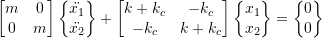The simplest approach is to look for Simultaneous Simple Harmonic Motion (SSHM). That is: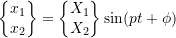Which is a solution (not the general one) if: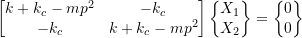This can be true if and only if (iff),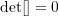. Therefore: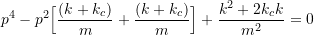There are two soloutions for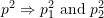.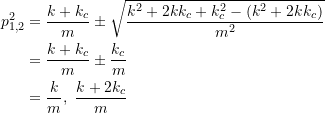Therefore: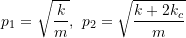As a result, there are 2 frequencies at which our assumption is true. NOTE: It turns out that the general solution can be determined from these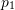,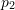, and the ratio of the amplitudes between the two masses during each of the two SSHMs. Therefore: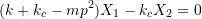Or: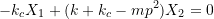Therefore, for either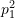or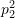: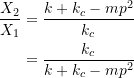If we putinto either we get: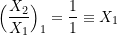And if we putinto either we get: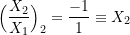Thus, we define the mode shapes corresponding to each ratio: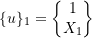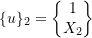Therefore, we have a mode shape corresponding to each of the natural frequencies.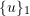and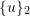are “normalized” vectors where the first component is set arbitrarily to unity. The general solution to the free vibration problem is then: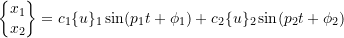There are 4 constants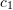,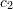,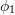,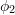for the 4 initial conditions for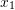,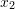,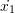,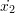. If the initial conditions are selected then the solution will include both modes in general but only one mode for certain cases. (For example, when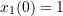,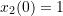,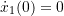, and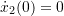,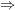mode 1).

Consider the following initial conditions: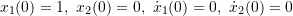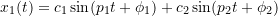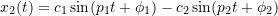At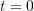: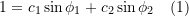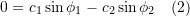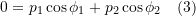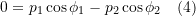Therefore: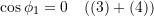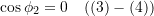Therefore: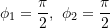From (1) and (2) (+):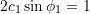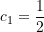From (1) and (2) (-):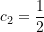Therefore: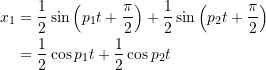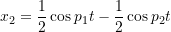Using identities: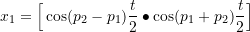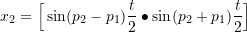These may be interpreted as a higher frequency oscillation at the average of the two natural frequencies with a variable amplitude, given by a lower frequency given bythe difference in natural frequencies.

Ifandare close to each relative to their magnitude then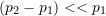, the motion becomes: